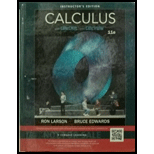Chapter 12.1, Problem 12E### Calculus (MindTap Course List)

11th Edition
Ron Larson + 1 other
ISBN: 9781337275347

#### Solutions

Chapter
Section### Calculus (MindTap Course List)

11th Edition
Ron Larson + 1 other
ISBN: 9781337275347
Textbook Problem

# Evaluating a function In Exercises 11 and 12 evaluate the vector-valued function at each given value of t. r ( t ) = cos   t i   +  2 sin  t j (a) r ( 0 ) . (b) r ( π / 4 ) (c) r ( θ − π ) (d) r ( π / 6 + Δ t ) − r ( π / 6 )

To determine

To calculate: The value of the vector function r(t)=costi+2sintj at each of the given value t.

r(0)

r( π4)

r (θπ)

r(π6+Δt)- r(π6)

Explanation

Given: Vector function: r(t)=costi+2sintj

r(0)

r (π4)

r (θπ)

r(π6+Δt)- r(π6)

Calculation:

Consider t as 0, π4,θ- π and π /6+Δt andπ/6 in order to determine the vector value:

r(0)=cos0i+2sin0j=i

r(π/4)=cosπ4i+2sinπ4j=12i+2j

r(θπ)=cos(θπ)i+2sin(θπ)j=cos[(πθ)]i+2sin[(πθ)]=cos[(πθ)]i2sin[(πθ)]j=cosθi

### Still sussing out bartleby?

Check out a sample textbook solution.

See a sample solution

#### The Answers to Your Study Problems

Solve them all with bartleby. Boost your grades with guidance from subject experts covering thousands of textbooks. All for just \$9.99/month

Get As ASAP

## Additional Math Solutions

#### Find more solutions based on key concepts